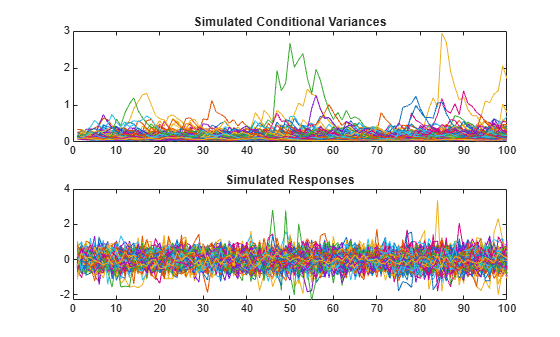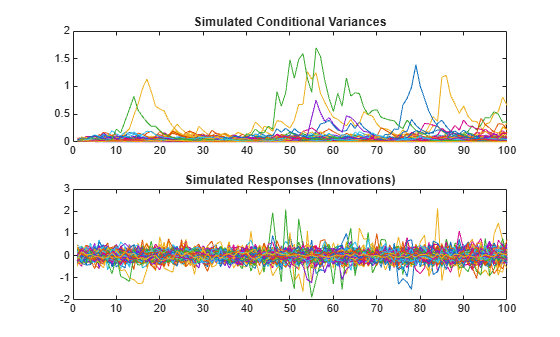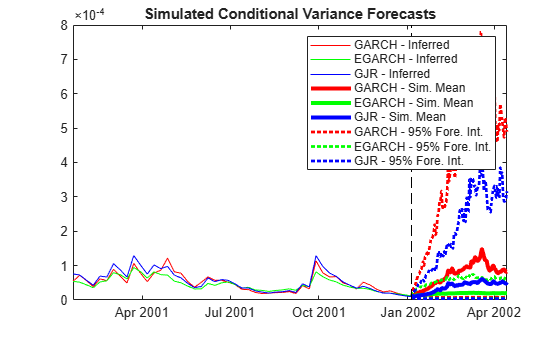# simulate

Monte Carlo simulation of conditional variance models

## Syntax

``V = simulate(Mdl,numobs)``
``V = simulate(Mdl,numobs,Name=Value)``
``````[V,Y] = simulate(___)``````
``Tbl = simulate(Mdl,numobs,Presample=Presample,Name=Value)``

## Description

example

````V = simulate(Mdl,numobs)` returns the numeric array `V` containing a random `numobs`-period path of a conditional variance series from the fully specified conditional variance model `Mdl`. `Mdl` can be a `garch`, `egarch`, or `gjr` model.```

example

````V = simulate(Mdl,numobs,Name=Value)` specifies options using one or more name-value arguments. For example, `simulate(Mdl,100,NumPaths=1000,V0=v0)` returns a numeric matrix of 1000, 100-period simulated conditional variance paths from `Mdl` and specifies the numeric vector of presample conditional variances `v0` to initialize each returned path.To produce a conditional simulation, specify response data in the simulation horizon by using the `YF` name-value argument.```

example

``````[V,Y] = simulate(___)``` also returns simulated response series paths using any of the input arguments in the previous syntaxes.```

example

````Tbl = simulate(Mdl,numobs,Presample=Presample,Name=Value)` returns the table or timetable `Tbl` containing the random conditional variance and response series, which results from simulating the model `Mdl`. `simulate` uses the table or timetable of innovations or conditional variance presample data `Presample` to initialize the model. If you specify `Presample`, you must specify the variable containing the presample innovation or conditional variance data by using the `PresampleInnovationVariable` or `PresampleVarianceVariable` name-value argument.```

## Examples

collapse all

Simulate conditional variance and response paths from a GARCH(1,1) model. Return results in numeric matrices.

Specify a GARCH(1,1) model with known parameters.

`Mdl = garch(Constant=0.01,GARCH=0.7,ARCH=0.2);`

Simulate 500 sample paths, each with 100 observations.

```rng("default") % For reproducibility [V,Y] = simulate(Mdl,100,NumPaths=500);```

`V` and `Y` are 100-by-500 matrices of 500 simulated paths of conditional variances and responses, respectively.

```figure tiledlayout(2,1) nexttile plot(V) title("Simulated Conditional Variances") nexttile plot(Y) title("Simulated Responses")```The simulated responses look like draws from a stationary stochastic process.

Plot the 2.5th, 50th (median), and 97.5th percentiles of the simulated conditional variances.

```lower = prctile(V,2.5,2); middle = median(V,2); upper = prctile(V,97.5,2); figure plot(1:100,lower,"r:",1:100,middle,"k", ... 1:100,upper,"r:",LineWidth=2) legend("95% Confidence interval","Median") title("Approximate 95% Intervals")```The intervals are asymmetric due to positivity constraints on the conditional variance.

Simulate conditional variance and response paths from an EGARCH(1,1) model.

Specify an EGARCH(1,1) model with known parameters.

```Mdl = egarch(Constant=0.001,GARCH=0.7,ARCH=0.2, ... Leverage=-0.3);```

Simulate 500 sample paths, each with 100 observations.

```rng("default") % For reproducibility [V,Y] = simulate(Mdl,100,NumPaths=500); figure tiledlayout(2,1) nexttile plot(V) title("Simulated Conditional Variances") nexttile plot(Y) title("Simulated Responses (Innovations)")```The simulated responses look like draws from a stationary stochastic process.

Plot the 2.5th, 50th (median), and 97.5th percentiles of the simulated conditional variances.

```lower = prctile(V,2.5,2); middle = median(V,2); upper = prctile(V,97.5,2); figure plot(1:100,lower,"r:",1:100,middle,"k", ... 1:100, upper,"r:",LineWidth=2) legend("95% Confidence interval","Median") title("Approximate 95% Intervals")```The intervals are asymmetric due to positivity constraints on the conditional variance.

Simulate conditional variance and response paths from a GJR(1,1) model.

Specify a GJR(1,1) model with known parameters.

```Mdl = gjr(Constant=0.001,GARCH=0.7,ARCH=0.2, ... Leverage=0.1);```

Simulate 500 sample paths, each with 100 observations.

```rng("default") % For reproducibility [V,Y] = simulate(Mdl,100,NumPaths=500); figure tiledlayout(2,1) nexttile plot(V) title("Simulated Conditional Variances") nexttile plot(Y) title("Simulated Responses (Innovations)")```The simulated responses look like draws from a stationary stochastic process.

Plot the 2.5th, 50th (median), and 97.5th percentiles of the simulated conditional variances.

```lower = prctile(V,2.5,2); middle = median(V,2); upper = prctile(V,97.5,2); figure plot(1:100,lower,"r:",1:100,middle,"k", ... 1:100, upper,"r:",LineWidth=2) legend("95% Confidence interval","Median") title("Approximate 95% Intervals")```The intervals are asymmetric due to positivity constraints on the conditional variance.

Since R2023a

Simulate conditional variances of the daily NASDAQ Composite Index returns for 500 days. Use the simulations to make forecasts and approximate 95% forecast intervals. Compare the forecasts among GARCH(1,1), EGARCH(1,1), and GJR(1,1) fits. Supply timetables of presample data.

Load the NASDAQ data included with the toolbox. Convert the index to returns.

```load Data_EquityIdx DTTRet = price2ret(DataTimeTable); DTTRet.Interval = []; T = height(DTTRet);```

Fit GARCH(1,1), EGARCH(1,1), and GJR(1,1) models to the entire data set.

```Mdl = cell(3,1); % Preallocation Mdl{1} = garch(1,1); Mdl{2} = egarch(1,1); Mdl{3} = gjr(1,1); EstMdl = cellfun(@(x)estimate(x,DTTRet,ResponseVariable="NASDAQ", ... Display="off"),Mdl,UniformOutput=false);```

`EstMdl` is 3-by-1 cell vector. Each cell is a different type of estimated conditional variance model, e.g., `EstMdl{1}` is an estimated GARCH(1,1) model.

Simulate 1000 samples paths with 500 observations each. Infer conditional variances and residuals to use as a presample for the forecast simulation.

```DTTSim = cell(3,1); % Preallocation PS = cell(3,1); for j = 1:3 rng("default") % For reproducibility PS{j} = infer(EstMdl{j},DTTRet,ResponseVariable="NASDAQ"); DTTSim{j} = simulate(EstMdl{j},500,NumPaths=1000, ... Presample=PS{j},PresampleInnovationVariable="Y_Residual", ... PresampleVarianceVariable="Y_Variance"); end```

`DTTSim` is a 3-by-1 cell vector, and each cell contains a 500-by-2 timetable of 1000 simulated paths of conditional variances and responses generated from the corresponding estimated model.

Plot the simulation mean forecasts and approximate 95% forecast intervals, along with the conditional variances inferred from the data.

```lower = cellfun(@(x)prctile(x.Y_Variance,2.5,2),DTTSim,UniformOutput=false); upper = cellfun(@(x)prctile(x.Y_Variance,97.5,2),DTTSim,UniformOutput=false); mn = cellfun(@(x)mean(x.Y_Variance,2),DTTSim,UniformOutput=false); datesPlot = DTTRet.Time(end - 250:end); datesFH = DTTRet.Time(end) + caldays(1:500)'; h = zeros(3,4); figure for j = 1:3 col = zeros(1,3); col(j) = 1; h(j,1) = plot(datesPlot,PS{j}.Y_Variance(end-250:end),Color=col); hold on h(j,2) = plot(datesFH,mn{j},Color=col,LineWidth=3); h(j,3:4) = plot([datesFH datesFH],[lower{j} upper{j}],":", ... Color=col,LineWidth=2); end hGCA = gca; plot(datesFH([1 1]),hGCA.YLim,"k--"); axis tight; h = h(:,1:3); legend(h(:),'GARCH - Inferred','EGARCH - Inferred','GJR - Inferred',... 'GARCH - Sim. Mean','EGARCH - Sim. Mean','GJR - Sim. Mean',... 'GARCH - 95% Fore. Int.','EGARCH - 95% Fore. Int.',... 'GJR - 95% Fore. Int.','Location','NorthEast') title('Simulated Conditional Variance Forecasts') hold off```## Input Arguments

collapse all

Conditional variance model without any unknown parameters, specified as a `garch`, `egarch`, or `gjr` model object.

`Mdl` cannot contain any properties that have `NaN` value.

Sample path length, specified as a positive integer. `numobs` is the number of random observations to generate per output path; the outputs `V` and `Y` have `numobs` rows.

Since R2023a

Presample data for innovations εt or conditional variances σt2 to initialize the model, specified as a table or timetable, the same type as `Tbl1`, with `numprevars` variables and `numpreobs` rows. Use `Presample` only when you supply a table or timetable of data `Tbl1`.

`simulate` returns the simulated variables in the output table or timetable `Tbl`, which is commensurate with `Presample`.

Each selected variable is a single path (`numpreobs`-by-1 vector) or multiple paths (`numpreobs`-by-`numprepaths` matrix) of `numpreobs` observations representing the presample of `numpreobs` observations of innovations or conditional variances.

Each row is a presample observation, and measurements in each row occur simultaneously. The last row contains the latest presample observation. `numpreobs` must be one of the following values:

• `Mdl.Q` when `Presample` provides only presample innovations.

• For GARCH(P,Q) and GJR(P,Q) models:

• `Mdl.P` when `Presample` provides only presample conditional variances.

• `max([Mdl.P Mdl.Q])` when `Presample` provides both presample innovations and conditional variances

• For EGARCH(P,Q) models, `max([Mdl.P Mdl.Q])` when `Presample` provides presample conditional variances

If `numpreobs` exceeds the minimum number, `simulate` uses the latest required number of observations only.

If `Presample` is a timetable, all the following conditions must be true:

• `Presample` must represent a sample with a regular datetime time step (see `isregular`).

• The datetime vector of sample timestamps `Presample.Time` must be ascending or descending.

If `Presample` is a table, the last row contains the latest presample observation.

The defaults are:

• For GARCH(P,Q) and GJR(P,Q) models, `simulate` sets any necessary presample innovations to the square root of the average squared value of the offset-adjusted response series `Y`.

• For EGARCH(P,Q) models, `simulate` sets any necessary presample innovations to zero.

• `simulate` sets any necessary presample conditional variances to the unconditional variance of the process.

If you specify the `Presample`, you must specify the presample innovation or conditional variance variable names by using the `PresampleInnovationVariable` or `PresampleVarianceVariable` name-value argument.

### Name-Value Arguments

Specify optional pairs of arguments as `Name1=Value1,...,NameN=ValueN`, where `Name` is the argument name and `Value` is the corresponding value. Name-value arguments must appear after other arguments, but the order of the pairs does not matter.

Before R2021a, use commas to separate each name and value, and enclose `Name` in quotes.

Example: `simulate(Mdl,100,NumPaths=1000,E0=[0.5; 0.5])` specifies generating `1000` sample paths of length 100 from the model `Mdl`, and using `[0.5; 0.5]` as the presample of innovations per path.

Number of sample paths to generate, specified as a positive integer. The outputs `V` and `Y` have `NumPaths` columns.

Example: `NumPaths=1000`

Data Types: `double`

Presample innovation paths εt, specified as a `numpreobs`-by-1 numeric column vector or a `numpreobs`-by-`numprepaths` matrix. Use `E0` only when you supply optional data inputs as numeric arrays.

The presample innovations provide initial values for the innovations process of the conditional variance model `Mdl`. The presample innovations derive from a distribution with mean 0.

`numpreobs` is the number of presample observations. `numprepaths` is the number of presample paths.

Each row is a presample observation, and measurements in each row occur simultaneously. The last row contains the latest presample observation. `numpreobs` must be at least `Mdl.Q`. If `numpreobs` > `Mdl.Q`, `simulate` uses the latest required number of observations only. The last element or row contains the latest observation.

• If `E0` is a column vector, it represents a single path of the underlying innovation series. `simulate` applies it to each output path.

• If `E0` is a matrix, each column represents a presample path of the underlying innovation series. `numprepaths` must be at least `NumPaths`. If `numprepaths` > `NumPaths`, `simulate` uses the first `NumPaths` columns only.

The defaults are:

• For GARCH(P,Q) and GJR(P,Q) models, `simulate` sets any necessary presample innovations to an independent sequence of disturbances with mean zero and standard deviation equal to the unconditional standard deviation of the conditional variance process.

• For EGARCH(P,Q) models, `simulate` sets any necessary presample innovations to an independent sequence of disturbances with mean zero and variance equal to the exponentiated unconditional mean of the logarithm of the EGARCH variance process.

Example: `E0=[0.5; 0.5]`

Positive presample conditional variance paths, specified as a `numpreobs`-by-1 positive column vector or `numpreobs`-by-`numprepaths` positive matrix.. `V0` provides initial values for the conditional variances in the model. Use `V0` only when you supply optional data inputs as numeric arrays.

Each row is a presample observation, and measurements in each row occur simultaneously. The last row contains the latest presample observation.

• For GARCH(P,Q) and GJR(P,Q) models, `numpreobs` must be at least `Mdl.P`.

• For EGARCH(P,Q) models,`numpreobs` must be at least `max([Mdl.P Mdl.Q])`.

If `numpreobs` exceeds the minimum number, `simulate` uses only the latest observations. The last element or row contains the latest observation.

• If `V0` is a column vector, it represents a single path of the conditional variance series. `simulate` applies it to each output path.

• If `V0` is a matrix, each column represents a presample path of the conditional variance series. `numprepaths` must be at least `NumPaths`. If `numprepaths` > `NumPaths`, `simulate` uses the first `NumPaths` columns only.

The defaults are:

• For GARCH(P,Q) and GJR(P,Q) models, `simulate` sets any necessary presample variances to the unconditional variance of the conditional variance process.

• For EGARCH(P,Q) models, `simulate` sets any necessary presample variances to the exponentiated unconditional mean of the logarithm of the EGARCH variance process.

Example: `V0=[1; 0.5]`

Data Types: `double`

Since R2023a

Variable of `Presample` containing presample innovation paths εt, specified as one of the following data types:

• String scalar or character vector containing a variable name in `Presample.Properties.VariableNames`

• Variable index (integer) to select from `Presample.Properties.VariableNames`

• A length `numprevars` logical vector, where `PresampleInnovationVariable(j) = true` selects variable `j` from `Presample.Properties.VariableNames`, and `sum(PresampleInnovationVariable)` is `1`

The selected variable must be a numeric matrix and cannot contain missing values (`NaN`).

If you specify presample innovation data by using the `Presample` name-value argument, you must specify `PresampleInnovationVariable`.

Example: `PresampleInnovationVariable="StockRateInnov0"`

Example: `PresampleInnovationVariable=[false false true false]` or `PresampleInnovationVariable=3` selects the third table variable as the presample innovation variable.

Data Types: `double` | `logical` | `char` | `cell` | `string`

Since R2023a

Variable of `Presample` containing data for the presample conditional variances σt2, specified as one of the following data types:

• String scalar or character vector containing a variable name in `Presample.Properties.VariableNames`

• Variable index (integer) to select from `Presample.Properties.VariableNames`

• A length `numprevars` logical vector, where `PresampleVarianceVariable(j) = true` selects variable `j` from `Presample.Properties.VariableNames`, and `sum(PresampleVarianceVariable)` is `1`

The selected variable must be a numeric vector and cannot contain missing values (`NaN`).

If you specify presample conditional variance data by using the `Presample` name-value argument, you must specify `PresampleVarianceVariable`.

Example: `PresampleVarianceVariable="StockRateVar0"`

Example: `PresampleVarianceVariable=[false false true false]` or `PresampleVarianceVariable=3` selects the third table variable as the presample conditional variance variable.

Data Types: `double` | `logical` | `char` | `cell` | `string`

Notes

• `NaN` values in `E0`, and `V0` indicate missing values. `simulate` removes missing values from specified data by list-wise deletion. `simulate` horizontally concatenates `E0` and `V0`, and then it removes any row of the concatenated matrix containing at least one `NaN`. This type of data reduction reduces the effective sample size and can create an irregular time series.

• For numeric data inputs, `simulate` assumes that you synchronize the presample data such that the latest observations occur simultaneously.

• `simulate` issues an error when any table or timetable input contains missing values.

• If `E0` and `V0` are column vectors, `simulate` applies them to every column of the outputs `V` and `Y`. This application allows simulated paths to share a common starting point for Monte Carlo simulation of forecasts and forecast error distributions.

## Output Arguments

collapse all

Simulated conditional variance paths σt2 of the mean-zero innovations associated with `Y`, returned as a `numobs`-by-1 numeric column vector or `numobs`-by-`NumPaths` matrix. `simulate` returns `V` when you do not specify the input table or timetable `Presample`.

Each column of `V` corresponds to a simulated conditional variance path. Rows of `V` are periods corresponding to the periodicity of `Mdl`.

Simulated response paths yt, returned as a `numobs`-by-1 numeric column vector or `numobs`-by-`NumPaths` matrix. `simulate` returns `Y` when you do not specify the input table or timetable `Presample`.

`Y` usually represents a mean-zero, heteroscedastic time series of innovations with conditional variances given in `V` (a continuation of the presample innovation series `E0`).

`Y` can also represent a time series of mean-zero, heteroscedastic innovations plus an offset. If `Mdl` includes an offset, then `simulate` adds the offset to the underlying mean-zero, heteroscedastic innovations so that `Y` represents a time series of offset-adjusted innovations.

Each column of `Y` corresponds to a simulated response path. Rows of `Y` are periods corresponding to the periodicity of `Mdl`.

Simulated conditional variance σt2 and response yt paths, returned as a table or timetable, the same data type as `Presample`. `simulate` returns `Tbl` only when you supply the input `Presample`.

`Tbl` contains the following variables:

• The simulated conditional variance paths, which are in a `numobs`-by-`NumPaths` numeric matrix, with rows representing observations and columns representing independent paths. Each path represents the continuation of the corresponding path of presample conditional variances in `Presample`. `simulate` names the simulated conditional variance variable in `Tbl` `responseName_Variance`, where `responseName` is `Mdl.SeriesName`. For example, if `Mdl.SeriesName` is `StockReturns`, `Tbl` contains a variable for the corresponding simulated conditional variance paths with the name `StockReturns_Variance`.

• The simulated response paths, which are in a `numobs`-by-`NumPaths` numeric matrix, with rows representing observations and columns representing independent paths. Each path represents the continuation of the corresponding presample innovations path in `Presample`. `simulate` names the simulated response variable in `Tbl` `responseName_Response`, where `responseName` is `Mdl.SeriesName`. For example, if `Mdl.SeriesName` is `StockReturns`, `Tbl` contains a variable for the corresponding simulated response paths with the name `StockReturns_Response`.

If `Tbl` is a timetable, the following conditions hold:

• The row order of `Tbl`, either ascending or descending, matches the row order of `Preample`.

• `Tbl.Time(1)` is the next time after `Presample(end)` relative the sampling frequency, and `Tbl.Time(2:numobs)` are the following times relative to the sampling frequency.

 Bollerslev, T. “Generalized Autoregressive Conditional Heteroskedasticity.” Journal of Econometrics. Vol. 31, 1986, pp. 307–327.

 Bollerslev, T. “A Conditionally Heteroskedastic Time Series Model for Speculative Prices and Rates of Return.” The Review of Economics and Statistics. Vol. 69, 1987, pp. 542–547.

 Box, G. E. P., G. M. Jenkins, and G. C. Reinsel. Time Series Analysis: Forecasting and Control. 3rd ed. Englewood Cliffs, NJ: Prentice Hall, 1994.

 Enders, W. Applied Econometric Time Series. Hoboken, NJ: John Wiley & Sons, 1995.

 Engle, R. F. “Autoregressive Conditional Heteroskedasticity with Estimates of the Variance of United Kingdom Inflation.” Econometrica. Vol. 50, 1982, pp. 987–1007.

 Glosten, L. R., R. Jagannathan, and D. E. Runkle. “On the Relation between the Expected Value and the Volatility of the Nominal Excess Return on Stocks.” The Journal of Finance. Vol. 48, No. 5, 1993, pp. 1779–1801.

 Hamilton, J. D. Time Series Analysis. Princeton, NJ: Princeton University Press, 1994.

 Nelson, D. B. “Conditional Heteroskedasticity in Asset Returns: A New Approach.” Econometrica. Vol. 59, 1991, pp. 347–370.